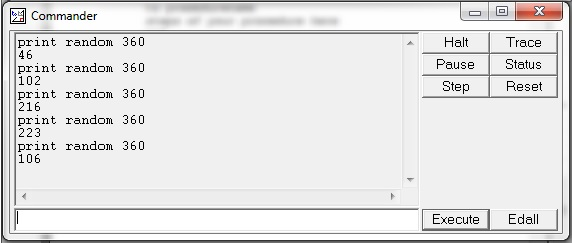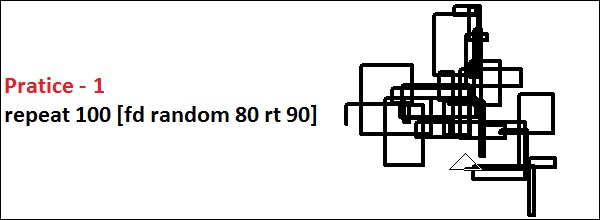# Logo - Randomization

Sometimes it is fun to have an unpredictable outcome of a computation. Logo provides a random procedure to generate a random number. It has one argument and produces an integer value chosen uniformly at random, which is greater than or equal to 0 and less than the value of its argument. Thus, if you want a random angle between 0 and 359 degrees, you can use the command random 360 to produce it. Bear in mind that Logo will show an error unless you do something with the result, such as printing.

Let us look at the following example −We have issued the command – print random 360 several times in the above command window and if you notice, each time it generates a random output.

Following are few practice commands with the desired results on the right.